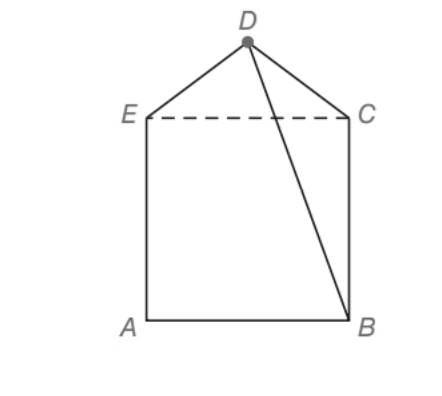Chapter 5.5, Problem 38E### Elementary Geometry for College St...

6th Edition
Daniel C. Alexander + 1 other
ISBN: 9781285195698

#### Solutions

Chapter
Section### Elementary Geometry for College St...

6th Edition
Daniel C. Alexander + 1 other
ISBN: 9781285195698
Textbook Problem
5 views

# Diagonal E C ¯ separates pentagon A B C D E into square A B C E and isosceles triangle D E C . If A B = 8 and D C = 5 , find the length of diagonal D B ¯ .(HINT: Draw D F ¯ ⊥ A B ¯ .)To determine

To find:

The length of diagonal DB¯ of a pentagon ABCDE such that diagonal EC¯ separates the pentagon ABCDE into a square ABCE and isosceles triangle DEC. AB=8 and DC=5.

Explanation

Approach:

Pythagorean Theorem.

In any right triangle, the square of the longer side is equal to the sum of the squares of the other two legs of the triangle.

For the right triangle XYZ with right angle at Z,

XY2=XZ2+YZ2.

Calculation:

Given,

A pentagon ABCDE with diagonal DB and EC.

ABCE is a square and DEC an isosceles triangle.

AB=8

DC=5

Since, ABCE is a square, all the four sides are congruent, and in the isosceles DEC, the sides DE and DC are congruent.

Thus,

AB=BC=CE=EA=8 units

DC=DE=5 units,

DF is the perpendicular drawn from D to AB.

The perpendicular DF bisects the square and the triangle into two congruent figures.

Now DGE is a right triangle with

DE=5= Hypotenuse,

EG=4= one of the leg

### Still sussing out bartleby?

Check out a sample textbook solution.

See a sample solution

#### The Solution to Your Study Problems

Bartleby provides explanations to thousands of textbook problems written by our experts, many with advanced degrees!

Get Started

#### Evaluate the integral. 17. e2sin3d

Single Variable Calculus: Early Transcendentals

#### True or False:

Study Guide for Stewart's Multivariable Calculus, 8th

#### True or False: xy + 6x2y = 10 x3 is linear.

Study Guide for Stewart's Single Variable Calculus: Early Transcendentals, 8th